Welcome to Doom9's Forum, THE in-place to be for everyone interested in DVD conversion. Before you start posting please read the forum rules. By posting to this forum you agree to abide by the rules.Doom9's ForumMVTools-pfmod
 Register FAQ Calendar Search Today's Posts Mark Forums Read8th November 2019, 03:26 #521  |  Link StainlessS HeartlessS Usurer   Join Date: Dec 2009 Location: Over the rainbow Posts: 7,216 Further to above, a bit more weirdness. Actually, weirdness on forward vectors when movement from right to left, but on backward vectors when movement left to right. Here script to show more masks over several frames. script (requires V.C. Mohan Grid plug if SHOWGRID=true) Code: ```MASKT=2 # 0, 2, 3 ONLY : 0=Motion, 2=Occlusion, 3=Horizontal motion HFLIP=true # Direction, true=Left to right XSTEP=8 # Movement step size per frame of white movement moving over black BLKSZ=4 # MAnalyse BlkSize Weird=true # Show Weird Occlusion masks SHOWGRID=true # Show Grid, Req V.C. Mohan Grid Plugins. ##################### ##### END Config #### ##################### CS="YV12" Wid=960 Hit=48 DELTA=2 TIME=50.0 Steps=Wid/XStep White=BlankClip(width=WID,height=HIT,Length=1,Color=\$FFFFFF,Pixel_type=CS) Black=White.BlankClip srcS=Black.BlankClip(Length=0) For(i=1,Steps-1) { W=White.Crop(0,0,i*XStep,0) K=Black.Crop(0,0,Wid-W.Width,0) Frm=StackHorizontal(K,W) srcS=srcS++Frm } srcS=(HFlip)?srcS.FlipHorizontal:srcS srcE=SrcS.Loop(0,0,Delta-1) # Delete Delta frames, end interp src OLap=(Weird)?2:Undefined super=srcS.MSuper(pel=2,hpad=16,vpad=16,chroma=false) fvec = MAnalyse(super, isb=false, blksize=BlkSz,Overlap=OLap,delta=Delta, truemotion=true,chroma=false) bvec = MAnalyse(super, isb=true, blksize=BlkSz,Overlap=OLap,delta=Delta, truemotion=true,chroma=false) inter= srcS.MFlowInter(super, bvec,fvec, time=Time).Greyscale mfv0 = srcS.MMask(fvec,kind=MaskT).Greyscale # n mfvS1= mfv0.DuplicateFrame(0) # n-1 mfv1 = mfv0.DeleteFrame(0) # n+1 mfv2 = mfv1.DeleteFrame(0) # n+2 mfv3 = mfv2.DeleteFrame(0) # n+3 mfv4 = mfv3.DeleteFrame(0) # n+4 mbv0 = srcS.MMask(bvec,kind=MaskT).Greyscale # n mbvS1= mbv0.DuplicateFrame(0) # n-1 mbv1 = mbv0.DeleteFrame(0) # n+1 mbv2 = mbv1.DeleteFrame(0) # n+2 mbv3 = mbv2.DeleteFrame(0) # n+3 mbv4 = mbv3.DeleteFrame(0) # n+4 DIR=(HFlip) ? " : ---->" : " : <----" TXT=SrcS.BlankClip(Height=20,Length=1,Color=\$404040).Subtitle(String(Delta,"Delta=%.0f")+String(Time," : Time=%.2f")+ \ String(BLKSZ," : BLKSZ=%.0f")+String(XSTEP," : XSTEP=%.0f")+" : HFlip="+String(HFlip)+DIR) StackVertical(srcS,SrcE,Inter,mfvS1,mbvS1,mfv0,mbv0,mfv1,mbv1,mfv2,mbv2,mfv3,mbv3,mfv4,mbv4) COL=\$FF00FF (SHOWGRID)?grid(Lineint=8,Color=COL,bColor=COL,vbColor=COL):NOP mType=mTyp(MaskT) ARGS = "Delta,Time,XStep,HFlip,MType" Last.GScriptClip("Fn(last, "+ARGS+")", local=true, args=ARGS) Return StackVertical(TXT,Last).ConvertToRGB32 Function MTyp(Int n) {Return " Type="+Select(n,"Motion","Sad","Occlusion","Horizontal","Vertical","ColorMap")} Function Fn(clip c,Int Delta,Float Time,Int XStep,Bool HFlip,String mType) { c n=current_frame Hit=Height/15 Steps=Width/XStep x=((n+1)*XStep) + (Delta*XStep/2) x=Min(x,(Steps-1)*XStep) x=HFlip?x:Width-1-x Subtitle(String(n,"SRC[n=%.0f] (1st interp srcS)") , Y=0.5*Hit,Align=5) Subtitle(String(n+Delta,"SRC[n+Delta=%.0f] (2nd interp srcE)") , Y=1.5*Hit,Align=5) Subtitle(string(n+Delta*Time/100.0,"MFlowInter Predicted @ [%.2f]") , Y=2.5*Hit,Align=5) Subtitle(String(n-1,"FVEC[n-1=%.0f]")+mType,Y=3.5*Hit,Align=5) Subtitle(String(n-1,"BVEC[n-1=%.0f]")+mType,Y=4.5*Hit,Align=5) Subtitle(String(n+0,"FVEC[n+0=%.0f]")+mType,Y=5.5*Hit,Align=5) Subtitle(String(n+0,"BVEC[n+0=%.0f]")+mType,Y=6.5*Hit,Align=5) Subtitle(String(n+1,"FVEC[n+1=%.0f]")+mType,Y=7.5*Hit,Align=5) Subtitle(String(n+1,"BVEC[n+1=%.0f]")+mType,Y=8.5*Hit,Align=5) Subtitle(String(n+2,"FVEC[n+2=%.0f]")+mType,Y=9.5*Hit,Align=5) Subtitle(String(n+2,"BVEC[n+2=%.0f]")+mType,Y=10.5*Hit,Align=5) Subtitle(String(n+3,"FVEC[n+3=%.0f]")+mType,Y=11.5*Hit,Align=5) Subtitle(String(n+3,"BVEC[n+3=%.0f]")+mType,Y=12.5*Hit,Align=5) Subtitle(String(n+4,"FVEC[n+4=%.0f]")+mType,Y=13.5*Hit,Align=5) Subtitle(String(n+4,"BVEC[n+4=%.0f]")+mType,Y=14.5*Hit,Align=5) Return Last }``` Here showing white motion coming in from the left. Mask=2, Occlusion (weirdness on backward vectors)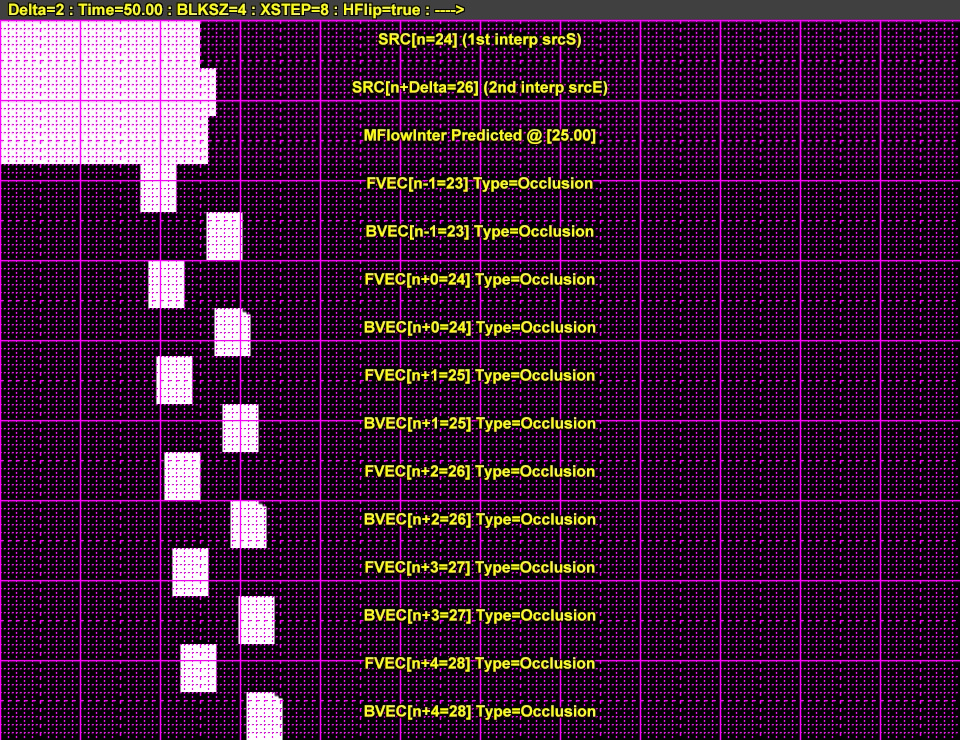Mask=0, Motion (its a bit faint) [relevant for frame n, bvec n+0 and fvec n+delta(2)]Mask=3, Horizontal Motion [relevant for frame n, bvec n+0 and fvec n+delta(2)]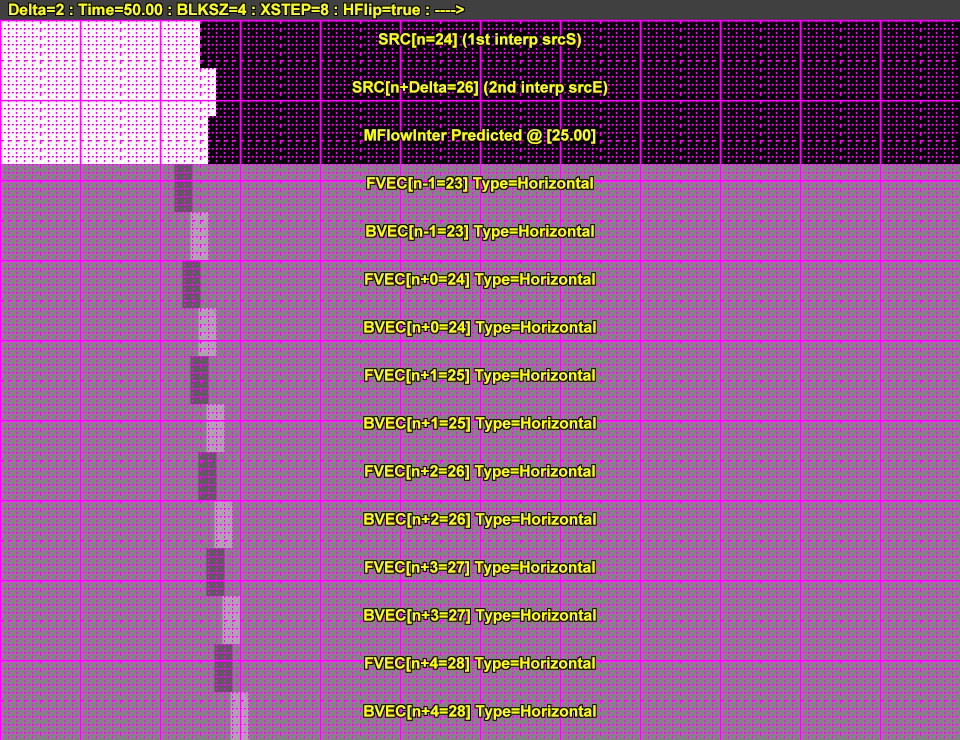Src frame 24 has stepped 2 * 8 pixels at frame 26, each little mark on grid are 8 pixels apart. __________________ I sometimes post sober. StainlessS@MediaFire ::: AND/OR ::: StainlessS@SendSpace "Some infinities are bigger than other infinities", but how many of them are infinitely bigger ??? Last edited by StainlessS; 11th November 2019 at 20:31.8th November 2019, 20:35 #522  |  Link manolito Registered User   Join Date: Sep 2003 Location: Berlin, Germany Posts: 2,633 Can you tell if only the pinterf-mod versions are affected by this "weirdness", or is it also present in the latest Fizick version mvtools-v2.5.11.22 ?9th November 2019, 10:17   #523  |  Link
StainlessS
HeartlessS Usurer

Join Date: Dec 2009
Location: Over the rainbow
Posts: 7,216
Thanks for taking an interest Mani,
And Yip, it does same in Avisynth v2.60, mvtools2 from mvtools-v2.5.11.22.zip (avs version 2.60 dll dated 26/Apr/2016).

just add Gscript wrapper as this [where not Avs+]

Code:
```GScript("""
For(i=1,Steps-1) {
W=White.Crop(0,0,i*XStep,0)  K=Black.Crop(0,0,Wid-W.Width,0)  Frm=StackHorizontal(K,W) srcS=srcS++Frm
}
""")```
Can you reproduce.

EDIT: I think the first script in post #520 should work as is (with Avs+ OR GScript, and at least version v2.60 standard, +Grid for 2nd script)

EDIT:
Quote:
 Originally Posted by StainlessSEDIT: I still dont have much clue how that occlusion stuff works. EDIT: I note that author of YFRC Code: ```# Author: RunForLife(Oleg Yushko) http://videomontazh.com.ua # http://avisynth.nl/index.php/YFRC``` uses only backwards Occlusion mask, here posted mod as YFRC2() by RaffRiff42:- https://forum.doom9.org/showthread.php?p=1804170 Below, both SAD masks used, but only BAK backward Occlusion mask. Code: ``` ## "EM" - error or artifact mask EM = C.MMask(bak, ml=190, kind=1) [** kind=SAD *] EM = EM.Overlay( \ C.MMask(fwd, ml=190, kind=1).DeleteFrame(0), \ opacity=0.5, mode="lighten") EM = EM.Overlay( \ C.MMask(bak, ml=64, kind=2).mt_inpand, [** kind=occlusion *] \ opacity=0.5, mode="lighten")```
I think above, that backward Occlusion mask should be mixed first with backward SAD mask, same for forward, and then those two mixed together.
(Dont know how MysteryX does it [EDIT: FrameRateConverter], I havnt looked)
__________________
I sometimes post sober.
StainlessS@MediaFire ::: AND/OR ::: StainlessS@SendSpace

"Some infinities are bigger than other infinities", but how many of them are infinitely bigger ???

Last edited by StainlessS; 9th November 2019 at 12:19.18th November 2019, 11:59 #524  |  Link pinterf Registered User   Join Date: Jan 2014 Posts: 1,247 When you visualize the vectors (a have put that line right after your vector generation lines, you can see that the forward vectors are not uniform at the top and right lines. Code: ``` [...] mbv=srcS.MMask(bvec,kind=MaskT) mfv=srcS.MMask(fvec,kind=MaskT) return StackVertical(MShow(super,fvec).SubTitle("MShow fvec"),MShow(super,bvec).SubTitle("MShow bvec"))``` Then change block size to 8, BlkSz=8, (default is 4 in your test script), then you can see other strange things18th November 2019, 19:05 #525  |  Link StainlessS HeartlessS Usurer   Join Date: Dec 2009 Location: Over the rainbow Posts: 7,216 Mod to VectorTest script as per Pinterf observation. Code: ```Function VectorTest(Int "Delta",Float "Time",Int "MaskT",Int "BlkSz",Int "XStep",Bool "Align",Bool "HFlip",String "CS") { /* VectorTest(), An MvTools2::MFlowInter folly. by StainlessS @ Doom9 : https://forum.doom9.org/showthread.php?t=175373 Req AVS+ or GSCript, GRunt, MvTools2, RT_Stats v1.43+ Avs v2.58, Avs/+ v2.60. Delta, Default 1. As for MvTools2 Time, Default 50.0, As for MvTools2 MaskT, Default 3, As for MvTools2::MMask(kind=MASK_TYPE), 0=Motion, 1=Sad, 2=Occlusion, 3=Horizontal, 4=Vertical, 5=ColorMap BlkSz, Default 4, As for MvTools2 XStep, Default 8, Motion per frame of synthesized clip. Align, Default True, Aligns forward vector frame n+Delta to n. False show frame n of forward vector. HFlip, Default False, False, Animate from Right to Left, else Left to Right. (Right to Left is less confusing, same direction that the frames come in from). CS, Avs v2.5 defaults "YV12" else "Y8". Returns RGB32 clip. */ Function MTyp(Int n) {Return " Type="+Select(n,"Motion","Sad","Occlusion","Horizontal","Vertical","ColorMap")} myName="VectorTest: " IsAvsPlus=(FindStr(UCase(versionString),"AVISYNTH+")!=0) HasGScript=RT_FunctionExist("GScript") HasGrunt=RT_FunctionExist("GScriptclip") HasMvTools2=RT_FunctionExist("MSuper") Is26=VersionNumber>=2.6 Assert(IsAvsPlus||HasGscript,myName+"Essential AVS+ or GScript installed") Assert(HasGrunt,myName+"Essential GRunt installed") Assert(HasMvTools2,myName+"Essential MvTools2 installed") Delta=Default(Delta,1) Time=Default(Time,50.0) MaskT=Default(MaskT,3) BlkSz=DefaulT(BlkSz,4) XStep=Default(XStep,8) Align=Default(Align,True) HFlip=Default(HFlip,False) CS=Default(CS,Is26?"Y8":"YV12") OLap=(BlkSz>=4)?BlkSz/2:RT_Undefined # OLap=RT_Undefined FuncS=""" Function Fn(clip c,Int Delta,Float Time,Int XStep,Bool Align,Bool HFlip,String mType) { c n=current_frame Hit=(Height-20)/5 if(Align) { Steps=640/XStep x=((n+1)*XStep) + (Delta*XStep/2) x=Min(x,(Steps-1)*XStep) x=HFlip?x:639-x cF=RT_YPlaneMin(n=n,x=x,y=Round(2.5*Hit)+20,w=1,h=1)-128 cB=RT_YPlaneMin(n=n,x=x,y=Round(3.5*Hit)+20,w=1,h=1)-128 Z=c.BlankClip(width=1,height=1,Color=\$FFFFFF,Length=1) OverLay(Z,x=x,y=Round(2.5*Hit)+20) OverLay(Z,x=x,y=Round(3.5*Hit)+20) Subtitle(RT_String("@x=%d FGrey=128%+d : BGrey=128%+d",x,cF,cB)) } else { Subtitle("Align=False, Colors NOT shown") } Subtitle(String(n,"SRC[n=%.0f] (1st interp srcS)") , Y=0.5*Hit+20,Align=5) Subtitle(String(n+Delta,"SRC[n+Delta=%.0f] (2nd interp srcE)") , Y=1.5*Hit+20,Align=5) (Align) \ ? Subtitle(String(n+Delta,"FVEC[n+DELTA=%.0f]")+mType+ " (Aligned)" , Y=2.5*Hit+20,Align=5) \ : Subtitle(String(n,"FVEC[n=%.0f]")+mType , Y=2.5*Hit+20,Align=5) Subtitle(String(n,"BVEC[n=%.0f]")+mType , Y=3.5*Hit+20,Align=5) Subtitle(string(n+Delta*Time/100.0,"MFlowInter Predicted @ [%.2f]") , Y=4.5*Hit+20,Align=5) Return Last } Wid=640 Hit=64 Steps=Wid/XStep White=BlankClip(width=WID,height=HIT,Length=1,Color=\$FFFFFF,Pixel_type=CS) Black=White.BlankClip srcS=Black.BlankClip(Length=0) For(i=1,Steps-1) { W=White.Crop(0,0,i*XStep,0) K=Black.Crop(0,0,Wid-W.Width,0) Frm=StackHorizontal(K,W) srcS=srcS++Frm } srcS=(HFlip)?srcS.FlipHorizontal:srcS srcE=SrcS.Loop(0,0,Delta-1) # Delete Delta frames, end interp src super=srcS.MSuper(pel=2,hpad=16,vpad=16) fvec =MAnalyse(super, isb=false, blksize=BlkSz, overlap=OLap, delta=Delta, truemotion=true) bvec =MAnalyse(super, isb=true, blksize=BlkSz, overlap=OLap, delta=Delta, truemotion=true) inter=srcS.MFlowInter(super, bvec,fvec, time=Time) mbv=srcS.MMask(bvec,kind=MaskT) mfv=srcS.MMask(fvec,kind=MaskT) return StackVertical(MShow(super,fvec).SubTitle("MShow fvec"),MShow(super,bvec).SubTitle("MShow bvec")) ### RETURN EARLY mfv=(Align)?mfv.Loop(0,0,Delta-1):mfv # Align, Delete DELTA frames from start, align n+delta with n DIR=(HFlip) ? " : ---->" : " : <----" TXT=SrcS.BlankClip(Height=20,Length=1,Color=\$404040) StackVertical(TXT,srcS,SrcE,MFV,MBV,Inter) mType=mTyp(MaskT) ARGS = "Delta,Time,XStep,Align,HFlip,MType" Last.GScriptClip("Fn(last, "+ARGS+")", local=true, args=ARGS) TXT=TXT.Subtitle(String(Delta,"Delta=%.0f")+String(Time," : Time=%.2f")+ \ String(BLKSZ," : BLKSZ=%.0f")+String(XSTEP," : XSTEP=%.0f")+" : ALIGN="+String(ALIGN)+" : HFlip="+String(HFlip)+DIR) return StackVertical(TXT,Last) """ IsAvsPlus?Eval(FuncS):GScript(FuncS) Return Last.ConvertToRGB32 } # 0=Motion, 1=Sad, 2=Occlusion, 3=Horizontal, 4=Vertical, 5=ColorMap VectorTest(Delta=2,MaskT=2,HFlip=false,BlkSz=8,Align=True)``` frame 41 [EDIT: BVEC looks correct-ish, but is it twice as wide as it should be ???, maybe white moving left, and black moving right, sort of ]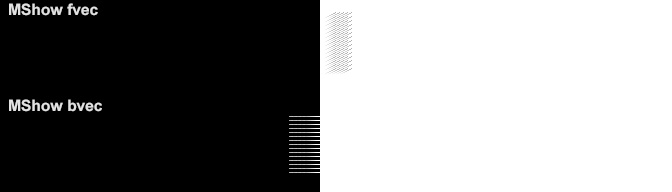frame 42frame 43frame 44frame 45 [EDIT: BVEC looks correct-ish, but is it twice as wide as it should be ???, maybe white moving left, and black moving right, sort of ]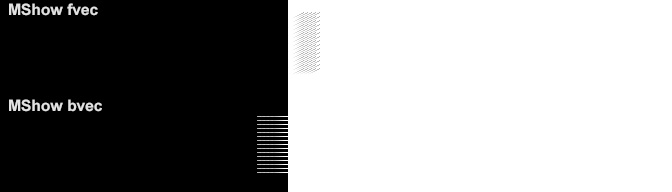'curiouser and curiouser' cried Alice. EDIT: Frame 41 (top one) cropped and point resized a bit Code: ```Crop(288,112,34,-14) PointResize(width*16,height*16)```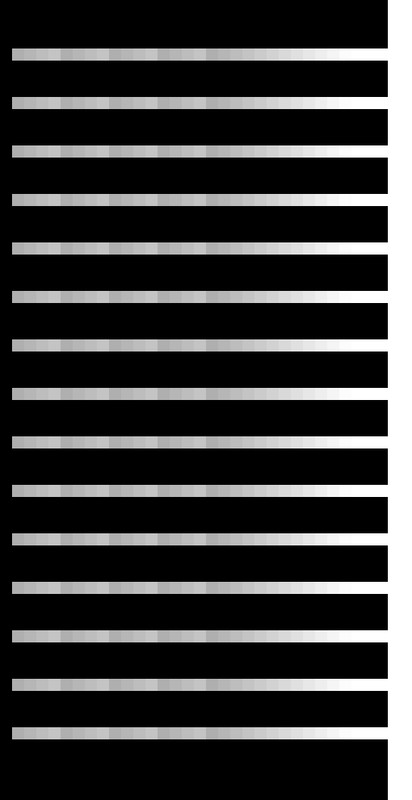__________________ I sometimes post sober. StainlessS@MediaFire ::: AND/OR ::: StainlessS@SendSpace "Some infinities are bigger than other infinities", but how many of them are infinitely bigger ??? Last edited by StainlessS; 18th November 2019 at 21:09.Thread Tools Search this ThreadShow Printable VersionEmail this Page Search this Thread: Advanced Search Display ModesLinear ModeSwitch to Hybrid ModeSwitch to Threaded ModePosting Rules You may not post new threads You may not post replies You may not post attachments You may not edit your posts BB code is On Smilies are On [IMG] code is On HTML code is Off Forum Rules
 Forum Jump User Control Panel Private Messages Subscriptions Who's Online Search Forums Forums Home Announcements and Chat     General Discussion     News     Forum / Site Suggestions & Help General     Decrypting     Newbies     DVD2AVI / DGIndex     Audio encoding     Subtitles     Linux, Mac OS X, & Co Capturing and Editing Video     Avisynth Usage     Avisynth Development     VapourSynth     Capturing Video     DV     HDTV / DVB / TiVo     NLE - Non Linear Editing     VirtualDub, VDubMod & AviDemux     New and alternative a/v containers Video Encoding     (Auto) Gordian Knot     MPEG-4 ASP     MPEG-4 Encoder GUIs     MPEG-4 AVC / H.264     High Efficiency Video Coding (HEVC)     New and alternative video codecs     MPEG-2 Encoding (HD) DVD, Blu-ray & (S)VCD     One click suites for DVD backup and DVD creation     DVD & BD Rebuilder     (HD) DVD & Blu-ray authoring     Advanced authoring     IFO/VOB Editors     DVD burning Hardware & Software     Software players     Hardware players     PC Hard & Software Programming and Hacking     Development     Translations

All times are GMT +1. The time now is 02:29.

 Doom9.org - Archive - Top